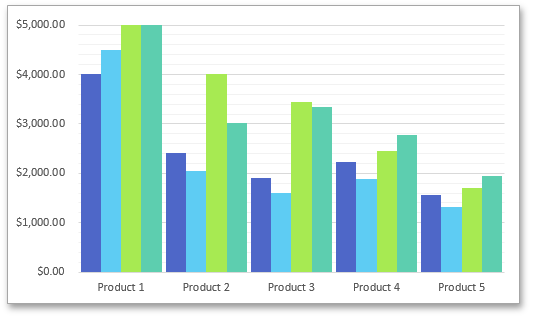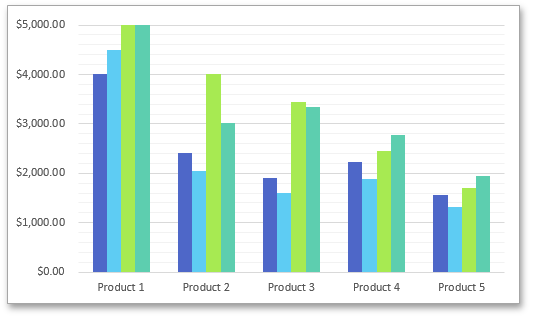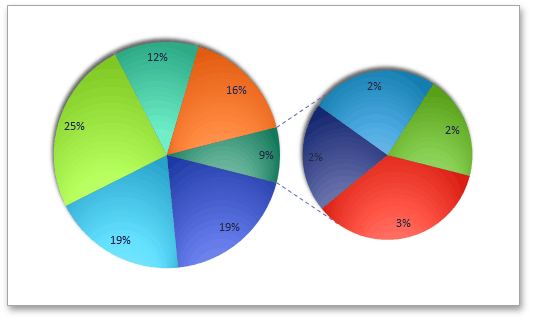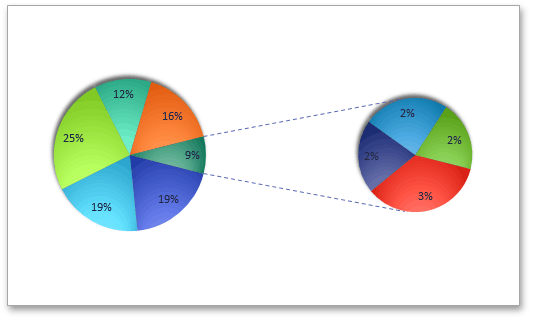20.2
20.1
19.2
19.1
18.2
18.1
17.2
.NET Framework 4.5.2+
.NET Framework 4.5.2+
.NET Standard 2.0+

# ChartView.GapWidth Property

Gets or sets the space between bar or column clusters in the Bar or Column charts, or the space between the primary and secondary charts in the Pie of Pie or Bar of Pie charts.

## Declaration

``int GapWidth { get; set; }``
``Property GapWidth As Integer``

## Property Value

Type Description
Int32

An integer value that is the percentage of the gap width. This value must be between 0 and 500.

## Remarks

Use the GapWidth property to control the space between each bar or column in the Bar or Column chart with one series, or the space between each group of bars or columns if the chart has multiple series.

Utilizing this property for the Pie of Pie or Bar of Pie chart changes the space between the primary and secondary charts.

The following images demonstrate how the GapWidth property works (the charts are shown in Microsoft® Excel®)

GapWidth = 20 GapWidth = 200## Examples

The example below demonstrates how to create the clustered column chart and specify the space between each column using the ChartView.GapWidth property.

``````Dim worksheet As Worksheet = workbook.Worksheets("chartTask5")
workbook.Worksheets.ActiveWorksheet = worksheet

' Create a chart and specify its location.
Dim chart As Chart = worksheet.Charts.Add(ChartType.ColumnClustered, worksheet("B2:C8"))
chart.TopLeftCell = worksheet.Cells("F2")
chart.BottomRightCell = worksheet.Cells("L15")

' Set the gap width between data series.
chart.Views(0).GapWidth = 33
' Hide the legend.
chart.Legend.Visible = False
``````